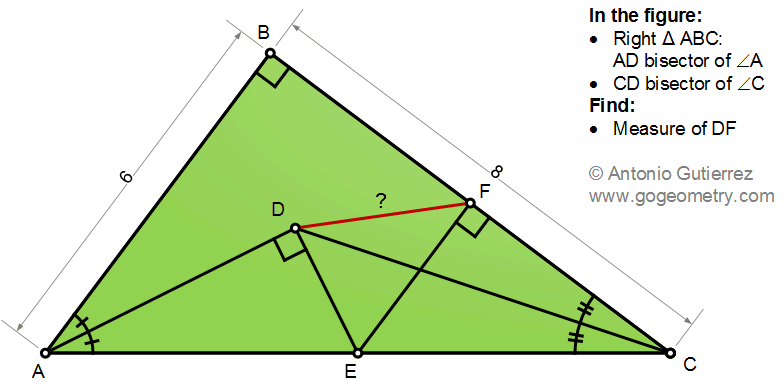# Geometry Problem 1489: Right Triangle, Angle Bisectors, Perpendicular, Measurement

The figure shows a right triangle ABC so that AD and CD are the bisectors of angles A and C. ED is perpendicular to AD and EF is perpendicular to BC. If AB = 6, and BC = 8, find the measure of DF.